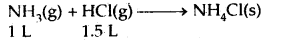## MCQ Quiz on Class 11 Chemistry Chapter 1 Some Basic Concepts of Chemistry with Answers

We have compiled the MCQ Questions for Class 11 Chemistry Chapter 1 Some Basic Concepts of Chemistry with Answers Pdf free download covering the entire syllabus for JEE/NEET and Boards. Practice MCQ Questions for Class 11 Chemistry with Answers on a daily basis and score well in exams. Refer to the Some Basic Concepts of Chemistry Class 11 MCQs Quiz Questions with Answers here along with a detailed explanation.

## Some Basic Concepts of Chemistry Class 11 MCQs Questions with Answers

Some Basic Concepts of Chemistry Class 11 MCQs Questions with Answers Explanation

1. Which of the following contains same number of carbon atoms as are in 6.0 g of carbon (C – 12)?

2. The prefix 1018 is

3. Which of the following cannot give iodometric titrations

4.  If we consider that 1/6, in place of 1/12, mass of carbon atom is taken to be the relative atomic mass unit, the mass of one mole of a substance will

5. The percentage of nitrogen in urea is about

6. What will be the volume of the mixture after the reaction7. The approximate atomic weight of an element is 26.89. If its equivalent weight is 8.9, the exact atomic weight of the element would be

8. The percentage of copper and oxygen in samples of CuO obtained by different methods were found to be the same. This illustrate the law of

9. The sulphate of a metal M contains 9.87% of M. This sulphate is isomorphous with ZnSO4.7H2O. The atomic weight of M is

10. How many mL of water must be added to 200 mL of .65 M HCL to dilute the solution to .20 M

11. What is the normality of a 1 M solution of H3PO4

12. The increasing order of molarity with 25 gm each of NaOH, LiOH, Al(OH)3, KOH, B(OH)3 in same volume of water?

13. Elements X and Y combine to form two compounds XY and X2Y. Find the atomic weight of X and Y, if the weight of 0.1 moles of XY is 10g and 0.05 moles of X2Y is 9g

14. Which of the following weighs the most?

15. For the reaction Fe2O3 + 3CO→ 2Fe + 3CO2, the volume of carbon monoxide required to reduce one mole of ferric oxide is

16.  Boron exists as two stable isotopes; 10B(19%) and 11B(81%). Find out the average atomic weight of boron in the periodic table

17. There are two chlorides of sulphur S2Cl2 and SCl2. What is the equivalent mass of S in SCl2

18. Number of atoms in 558.6 g Fe (Molar mass Fe = 55.86 g mol-1) is

19.  A container has an equal mass of H2, Oand CH4 at 27℃, the ratio of their volume is

20. A symbol not only represents the name of the element but also represents

Hope the information shed above regarding MCQ Questions for Class 11 Chemistry Chapter 1 Some Basic Concepts of Chemistry with Answers Pdf free download has been useful to an extent. If you have any other queries of CBSE Class 11 Chemistry Some Basic Concepts of Chemistry MCQs Multiple Choice Questions with Answers, feel free to comment below so that we can revert back to us at the earliest possible.

By Team Study Rate

This site uses Akismet to reduce spam. Learn how your comment data is processed.